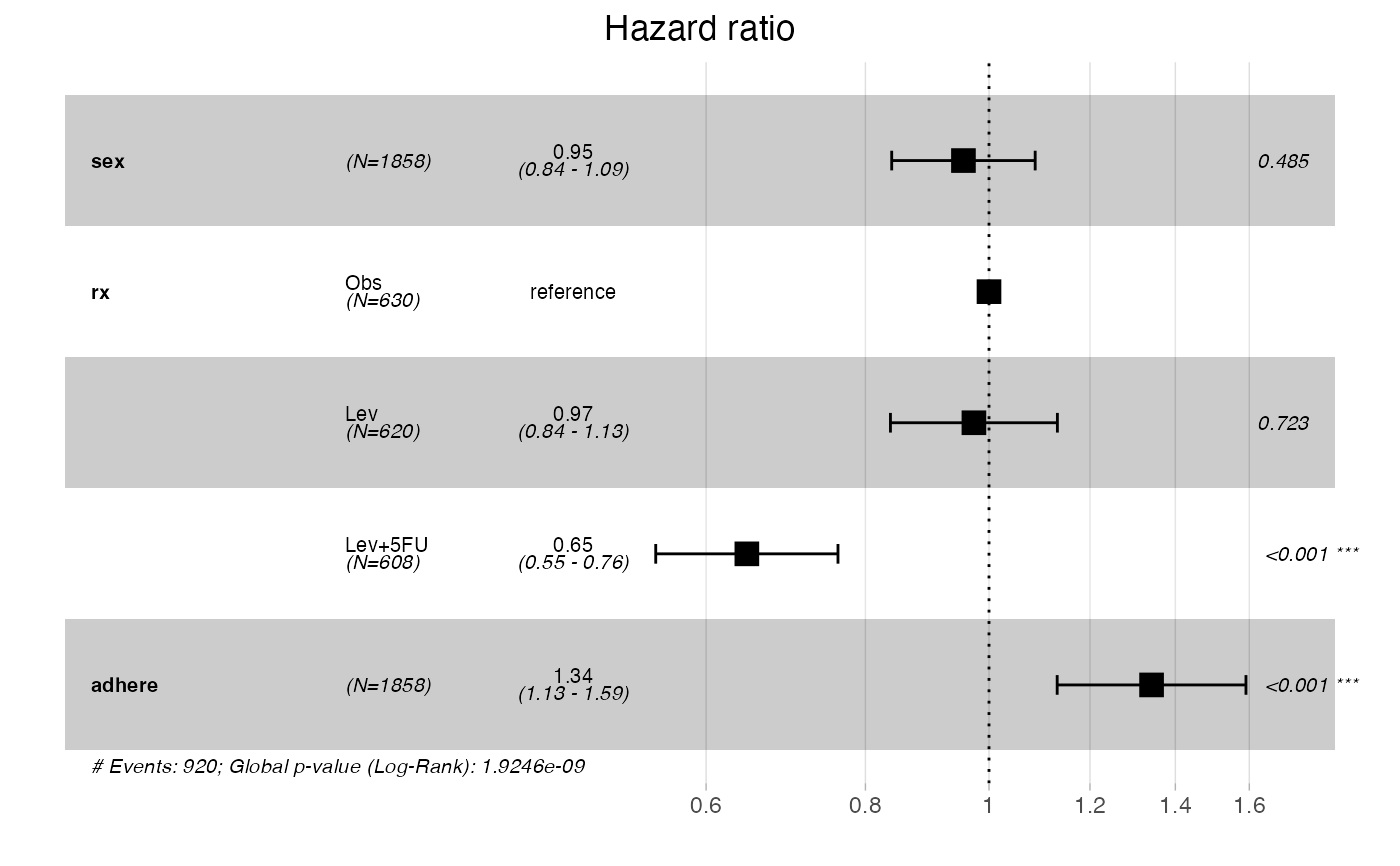Drawing Forest Plot for Cox proportional hazards model. In two panels the model structure is presented.

ggforest(
model,
data = NULL,
main = "Hazard ratio",
cpositions = c(0.02, 0.22, 0.4),
fontsize = 0.7,
refLabel = "reference",
noDigits = 2
)

Arguments

model an object of class coxph. a dataset used to fit survival curves. If not supplied then data will be extracted from 'fit' object. title of the plot. relative positions of first three columns in the OX scale. relative size of annotations in the plot. Default value: 0.7. label for reference levels of factor variables. number of digits for estimates and p-values in the plot.

Value

returns a ggplot2 object (invisibly)

Examples

require("survival") model <- coxph( Surv(time, status) ~ sex + rx + adhere, data = colon ) ggforest(model)
#> Warning: The data argument is not provided. Data will be extracted from model fit.colon <- within(colon, { sex <- factor(sex, labels = c("female", "male")) differ <- factor(differ, labels = c("well", "moderate", "poor")) extent <- factor(extent, labels = c("submuc.", "muscle", "serosa", "contig.")) }) bigmodel <- coxph(Surv(time, status) ~ sex + rx + adhere + differ + extent + node4, data = colon ) ggforest(bigmodel)
#> Warning: The data argument is not provided. Data will be extracted from model fit.